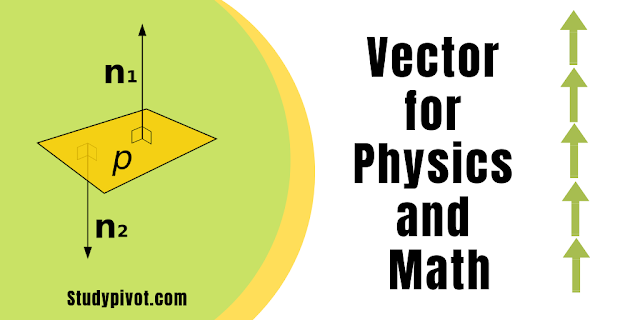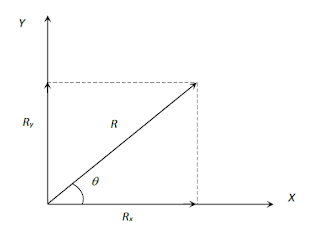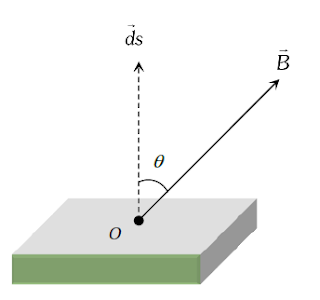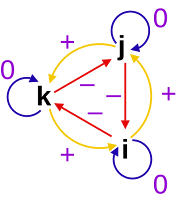# Vectors Tutorial for Physics and Math

## Vectors for Physics and MathIn this Vectors Physics and Math tutorial you will learn vectors definition, Types of Vector, Triangle Law of Vector Addition of Two Vectors, Parallelogram Law of Vector Addition, Polygon Law of Vector Addition, vector subtraction, Resolution of Vector Into Components, scalar multiplication (Dot Product), vector multiplication (Cross Product), vector algebra, Lami’s Theorem, Relative Velocity, concepts for finding vectors with some vector examples and illustrations. Vector is one of the most important topics for Physics and mathematics

## What is a Vector?

Physical quantities having magnitude, direction and obeying laws of vector algebra is called vector.
Example: Displacement, velocity, acceleration, momentum, force, impulse, weight, thrust, torque, angular momentum, angular velocity etc. are vector quantities.
If a physical quantity has magnitude and direction both, then it does not always imply that it is a vector. For it to be a vector the third condition of obeying laws of vector algebra has to be satisfied.
Example: The physical quantity current has both magnitude and direction but is still a scalar as it disobeys the laws of vector algebra.

## UPDATED LINK FOR MATHEMATICS COMPLETE NOTES

Examples, Theory and Formulas, Practice
Problems and worksheets of all topics of
Mathematics.

### Types of Vector

(1) Equal vectors: Two vectors $\overrightarrow{A}$ and $\overrightarrow{B}$ are said to be equal when they have equal magnitudes and same direction.

(2) Parallel vectors: Two vectors $\overrightarrow{A}$ and $\overrightarrow{B}$ are said to be parallel when
(i) Both have the same direction.
(ii) One vector is scalar (positive) non-zero multiple of another vector.

(3) Anti-parallel vectors: Two vectors $\overrightarrow{A}$ and $\overrightarrow{B}$ are said to be anti-parallel when
(i) Both have opposite direction.
(ii) One vector is a scalar non-zero negative multiple of another vector.

(4) Collinear vectors: When the vectors under consideration can share the same support or have common support then the considered vectors are collinear.

(5) Zero vector: $(\overrightarrow{0})$: A vector having zero magnitude and arbitrary direction (not known to us) is a zero vector.

(6) Unit vector: A vector divided by its magnitude is a unit vector. Unit vector for $\overrightarrow{A}$ is $\hat{A}$ (read as A cap or A hat).
Since, $\hat{A}=\frac{\overrightarrow{A}}{A}$ $\Rightarrow$$\overrightarrow{A}=A\,\hat{A}$.
Thus, we can say that the unit vector gives us direction.
Note: Here A is the modulus of vector A i.e |A|

(7) Orthogonal unit vectors: $\hat{i}\,,\,\hat{j}$ and $\hat{k}$ are called orthogonal unit vectors. These vectors must form a Right Handed Triad (It is a coordinate system such that when we Curl the fingers of the right hand from x to y then we must get the direction of z along thumb).$\hat{i}=\frac{\overrightarrow{x}}{x}$,$\hat{j}=\frac{\overrightarrow{y}}{y}$,$\hat{k}=\frac{\overrightarrow{z}}{z}$

\ $\overrightarrow{x}=x\hat{i}$, $\overrightarrow{y}=y\hat{j}$,
$\overrightarrow{z}=z\hat{k}$

(8) Polar vectors: These have a starting point or point of application. Example displacement and force etc.

(9) Axial Vectors: These represent rotational effects and are always along the axis of rotation in accordance with the right-hand screw rule. Angular velocity, torque and angular momentum, etc., are the example of physical quantities of this type.

(10) Co-planar vector: Three (or more) vectors are called coplanar vector if they lie in the same plane. Two (free) vectors are always co-planar.

### Triangle Law of Vector Addition of Two VectorsIf two non zero vectors are represented by the two sides of a triangle taken in the same order then the resultant is given by the closing side of the triangle in the opposite order.### Parallelogram Law of Vector Addition

If two non zero vectors are represented by the two adjacent sides of a parallelogram then the resultant is given by the diagonal of the parallelogram passing through the point of intersection of the two vectors.### Polygon Law of Vector AdditionIf a number of non zero vectors are represented by the (n – 1) sides of an n-sided polygon then the resultant is given by the closing side or the nth side of the polygon taken in the opposite order.

Note: 1. Resultant of two unequal vectors cannot be zero.

2. Resultant of three co-planar vectors may or may not be zero

3. Resultant of three non-coplanar vectors cannot be zero.

### Vector Subtraction### Resolution of Vector Into Components### Scalar Multiplication (Dot Product) of Two Vectors(1) Definition: The scalar multiplication (or dot product) of two vectors is defined as the product of the magnitude of two vectors with the cosine of the angle between them.### Vector Multiplication (Cross Product) of Two Vectors(1) Definition: The vector multiplication or cross product of two vectors is defined as a vector having a magnitude equal to the product of the magnitudes of two vectors with the sine of the angle between them, and direction perpendicular to the plane containing the two vectors in accordance with right-hand screw rule.

(2) Properties
(i) Vector product of any two vectors is always a vector perpendicular to the plane containing these two vectors, i.e., orthogonal(3) Example: Since vector product of two vectors is a vector, vector physical quantities (particularly representing rotational effects) like torque, angular momentum, velocity and force on a moving charge in a magnetic field and can be expressed as the vector product of two vectors. It is well – established in physics that :

### Lami’s Theorem### Relative Velocity

(1) Introduction: When we consider the motion of a particle, we assume a fixed point relative to which the given particle is in motion. For example, if we say that water is flowing or wind is blowing or a person is running with a speed v, we mean that these all are relative to the earth (which we have assumed to be fixed).(2) General Formula :

(3) The relative velocity of the satellite :

(5) The relative velocity of swimmer :

(6) Crossing the river :

### 3 thoughts on “Vectors Tutorial for Physics and Math”

1.cross product of two vectors pdf 2019

1.